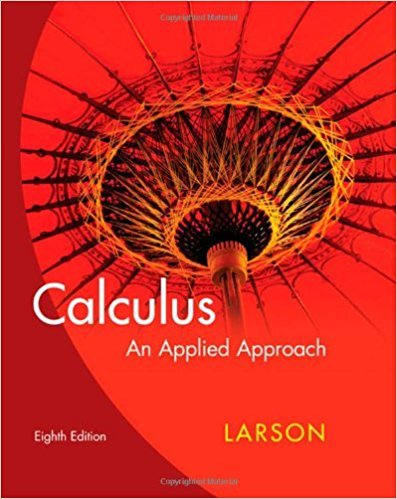×
×

# Solutions for Chapter 8.4: Derivatives of Trigonometric Functions## Full solutions for Calculus: An Applied Approach | 8th Edition

ISBN: 9780618958252Solutions for Chapter 8.4: Derivatives of Trigonometric Functions

Solutions for Chapter 8.4
4 5 0 347 Reviews
11
2
##### ISBN: 9780618958252

Chapter 8.4: Derivatives of Trigonometric Functions includes 87 full step-by-step solutions. Since 87 problems in chapter 8.4: Derivatives of Trigonometric Functions have been answered, more than 24020 students have viewed full step-by-step solutions from this chapter. This expansive textbook survival guide covers the following chapters and their solutions. Calculus: An Applied Approach was written by and is associated to the ISBN: 9780618958252. This textbook survival guide was created for the textbook: Calculus: An Applied Approach , edition: 8.

Key Calculus Terms and definitions covered in this textbook

If u = v and w = z , then u + w = v + z

• Blocking

A feature of some experimental designs that controls for potential differences between subject groups by applying treatments randomly within homogeneous blocks of subjects

• Circle graph

A circular graphical display of categorical data

• Coefficient matrix

A matrix whose elements are the coefficients in a system of linear equations

• Complex number

An expression a + bi, where a (the real part) and b (the imaginary part) are real numbers

• Cube root

nth root, where n = 3 (see Principal nth root),

• Directed angle

See Polar coordinates.

• Direction angle of a vector

The angle that the vector makes with the positive x-axis

• Equation

A statement of equality between two expressions.

• Horizontal Line Test

A test for determining whether the inverse of a relation is a function.

• Line of symmetry

A line over which a graph is the mirror image of itself

• Odd function

A function whose graph is symmetric about the origin (ƒ(-x) = -ƒ(x) for all x in the domain of f).

• Position vector of the point (a, b)

The vector <a,b>.

• Reference triangle

For an angle ? in standard position, a reference triangle is a triangle formed by the terminal side of angle ?, the x-axis, and a perpendicular dropped from a point on the terminal side to the x-axis. The angle in a reference triangle at the origin is the reference angle

• Reflexive property of equality

a = a

• Remainder polynomial

See Division algorithm for polynomials.

• Slant asymptote

An end behavior asymptote that is a slant line

• Slant line

A line that is neither horizontal nor vertical

• Translation

See Horizontal translation, Vertical translation.

• Transpose of a matrix

The matrix AT obtained by interchanging the rows and columns of A.

×